### AB PLC Data Types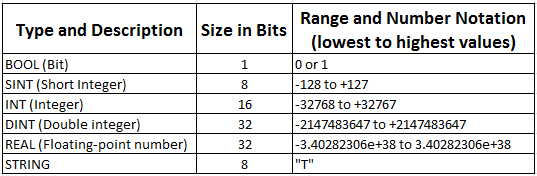### BOOL (Bit)

A Boolean variable, one whose value is either true or false.

Example:- 0 or 1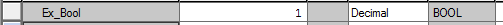### SINT (Short Integer)

Short Integer uses only 8 bits of memory and therefore is a value of 0 to 255 or if signed from -128 to +127.

Example:-  56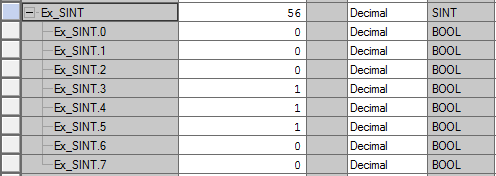### INT (Integer)

Integer uses only 16 bits of memory and therefore is a value of 0 to 65535 or if signed from -32768 to +32767.

Example:- 26733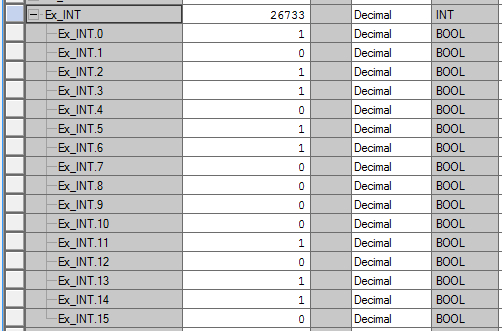### DINT (Double integer)

Double Integer uses only 32 bits of memory and therefore is a value of 0 to 4294967295 or if signed from -2147483647 to +2147483647.

Example:- 1436353644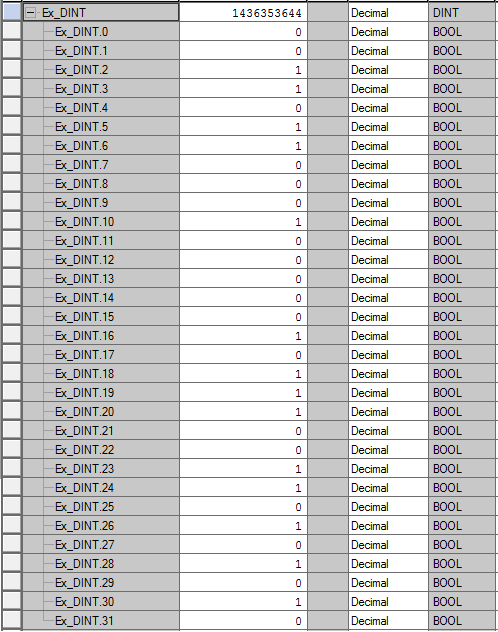### REAL (Floating-point number)

Real uses only 32 bits of memory. Value from -3.40282306e+38  to 3.40282306e+38

Example:-   -637.74646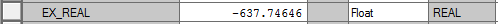### STRING

String uses only 8 bits of memory for single character. If you use 4 character total uses only 32 bits of memory

Example:-   "Test Word
T          - 8 bits
e           - 8 bits
s           - 8 bits
t            - 8 bits
Space   - 8 bits
W         - 8 bits
o           - 8 bits
r            - 8 bits
d           - 8 bits

Total     - 72 bits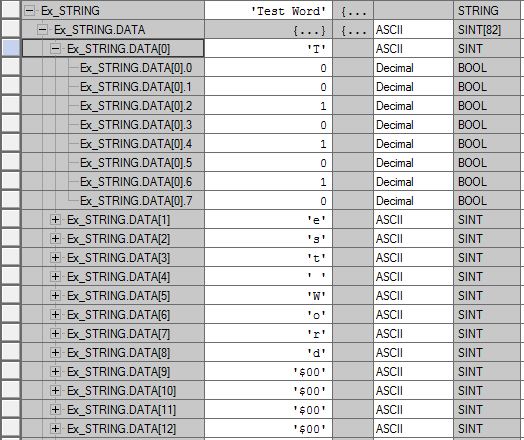#### Example from Rslogix 5000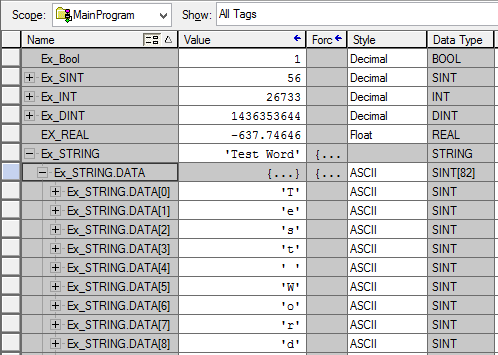### Convert 0-10 V to 0-20 mA Using Resistor

It is extremely simple to measure 0-10Vdc signal with a device that will measure only Current inputs. If Current input module available will accept a 0-20mA signal, but may not accept a 0-10Vdc signal directly.
Basically, Ohms law is used to calculate a resistor value in order to convert the 0-10Vdc signal to a current.
Voltage to Current Conversion Circuit Diagram

#### Example (0-10 VDC to 0-20 mA)

Ohms law states: R = V/I where V is the Voltage, I is the current and R is the resistance

R = 10V/0.020A = 500 Ohms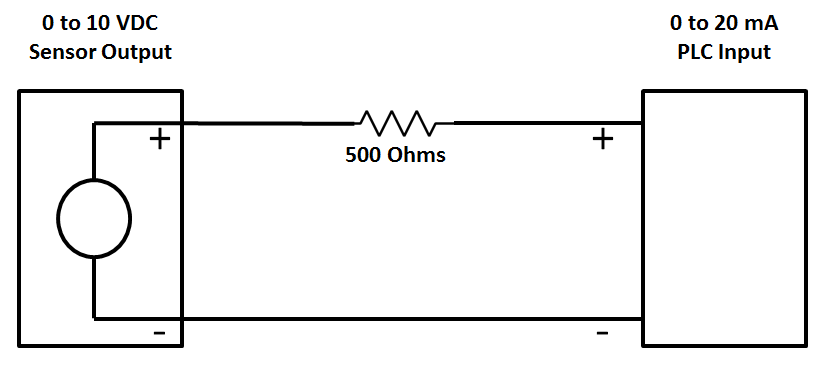0-10 VDC to 0-20 mA Conversion Circuit Diagram

I= V/R = 0/500   = 0mA

I= V/R = 10/500 = 0.02A = 20mA

#### Example (0-5 VDC to 0-20 mA)

Ohms law states: R = V/I where V is the Voltage, I is the current and R is the resistance

R = 5V/0.020A = 250 Ohms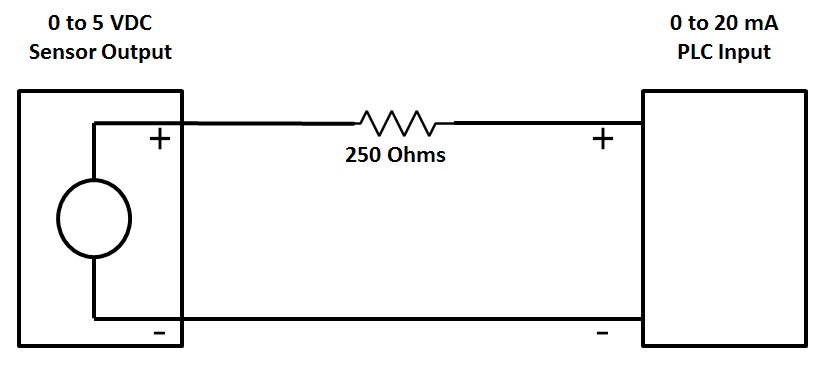0-5 VDC to 0-20 mA Conversion Circuit Diagram

I= V/R = 0/250   = 0mA

I= V/R = 5/250 = 0.02A = 20mA

#### Note:-

To avoid damage you must ensure that the external current source has short-circuit protection in all conductor cases.
The external resistor is a source of error because of its dependency on temperature and its inaccuracy.
In order to obtain measuring results that are as precise as possible it is recommended to use resistors with tolerances that are as small as possible.